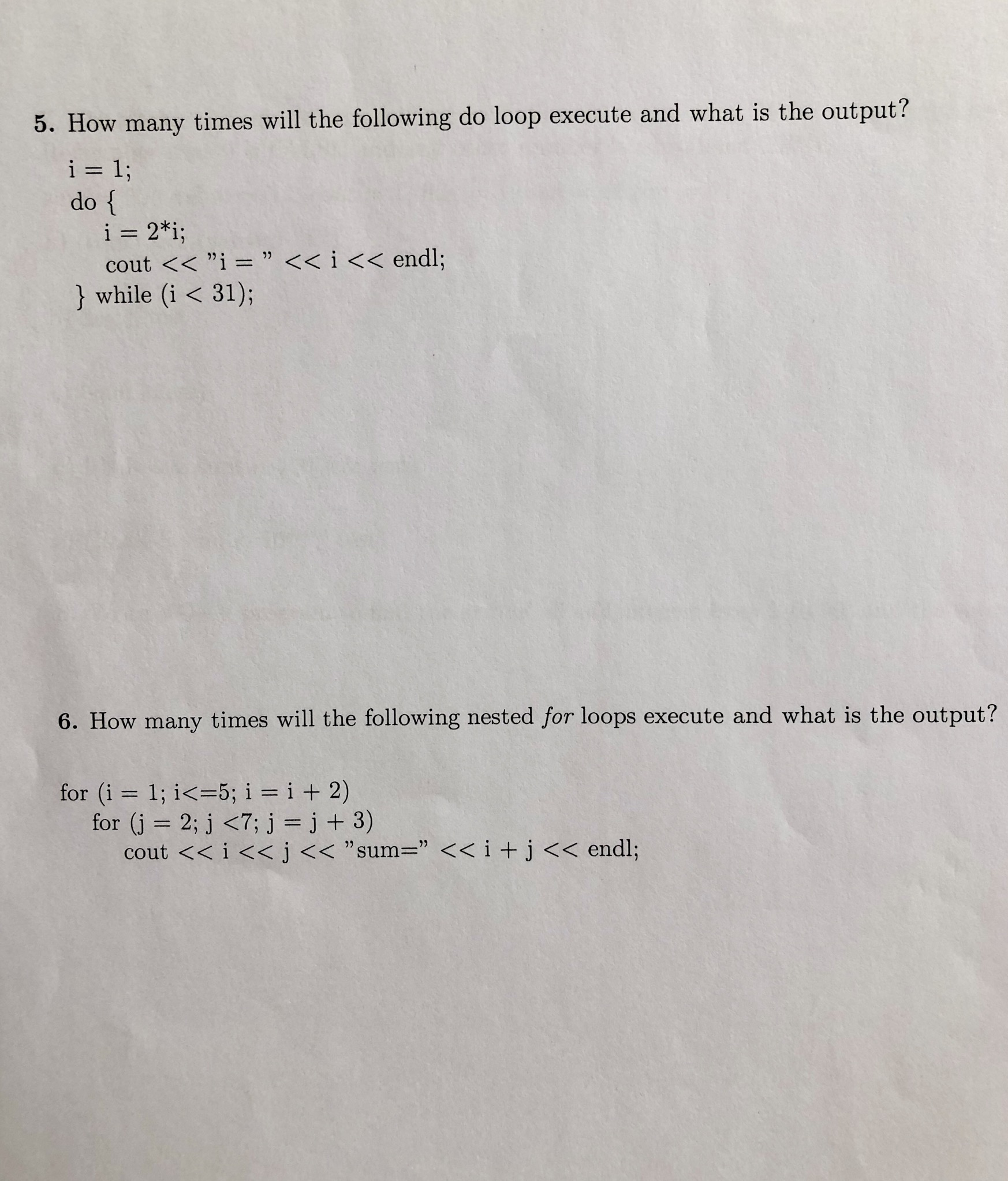# 5. How many times will the following do loop execute and what is the output?i 1;do {i= 2*i;cout <

Questionhelp_outlineImage Transcriptionclose5. How many times will the following do loop execute and what is the output? i 1; do { i= 2*i; cout <<"i = " << i << endl; } while (i < 31); 6. How many times will the following nested for loops execute and what is the out put ? for (i 1; i<-5; i i + 2) for (j 2; j <7; j = j + 3) cout << i <
check_circleExpert Solution

Determining the number of times the loop executes:

The loop will execute for 5 times. The “do..while” is the post check loop as the condition is evaluated after executing the statement inside the block. After executing ...

### Want to see the full answer?

See Solution

#### Want to see this answer and more?

Solutions are written by subject experts who are available 24/7. Questions are typically answered within 1 hour*

See Solution
*Response times may vary by subject and question
Tagged in

### Computer Science